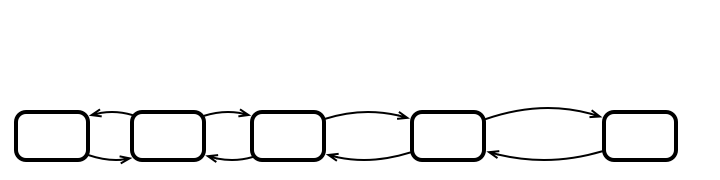Lecture 06: Concurrent Objects 1

Outline

1. Finishing Up Mutex:
• Bakery Algorithm
• Lower Bounds
2. Concurrent Objects

Last Time

Lamport’s Bakery Algorithm

public void lock () {
flag[i] = true;
label[i] = max(label, ..., label[n-1]) + 1;
while (!hasPriority(i)) {} // wait
}

public void unlock() {
}

We Showed:

• unique thread with highest priority obtains lock
2. First-come-first-served (FCFS) Property
• if i writes label[i] before j writes label[j], then i obtains lock before j

Starvation-freedom follows from 1 and 2

To Show: Mutual Exclusion

public void lock () {
flag[i] = true;
label[i] = max(label, ..., label[n-1]) + 1;
while (!hasPriority(i)) {} // wait
}

Suppose not:

• $A$ and $B$ concurrently in CS
• Assume: $(\mathrm{label}(A), A) < (\mathrm{label}(B), B)$

Proof (Continued)

Since $B$ entered CS:

• $(\mathrm{label}(B), B) < (\mathrm{label}(A), A)$, or
• $\mathrm{flag}[A] == \mathrm{false}$
• Former can not happen: labels strictly increasing

• So $B$ read $\mathrm{flag}[A] == \mathrm{false}$

Conclusion

Lamport’s Bakery Algorithm:

1. Works for any number of threads
2. Satisfies MutEx and starvation-freedom

Question

Is the bakery algorithm practical?

• label array contains $n$ indices
• must read all entries to set own label
• Could we do better?

Remarkably

We cannot do better:

• If $n$ threads want to achieve mutual exclusion + deadlock-freedom, must have $n$ read/write registers (variables)

• This is really bad if we have a lot of threads!
• 1,000 threads means each call to lock() requires 1,000s of reads
• each call to hasPriority requires either 1,000 of reads or a more advanced data structure
• Things are messy!

A Way Around the Lower Bound

• Argument relies crucially on fact that the only atomic operations are read and write
• Modern computers offer more powerful atomic operations
• In Java, AtomicBoolean class offers, e.g.,
• compareAndSet(boolean expectedValue, boolean newValue)
• getAndSet(boolean newValue)
• These operations are useful, but still costly
• We will discuss more later

Concurrent Objects

So Far

Considered mutual exclusion:

• This makes sense for simple objects
• e.g., the increment() method in CounterInsertion 1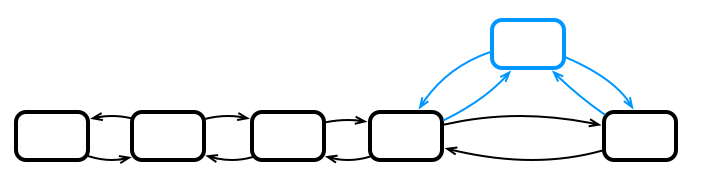Insertion 2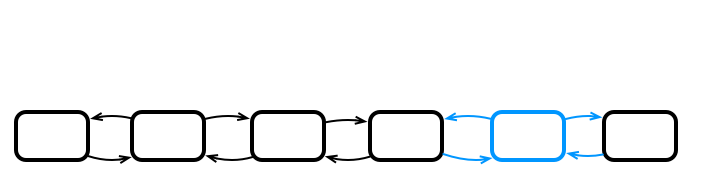...

// insert a new node after nd
public void insert (Node nd, value) {
Node next = nd.getNext();
Node cur = new Node(value);
nd.next = cur;
cur.prev = nd;
cur.next = next;
if (next != null) next.prev = cur;
}
}

class Node {
public Node next;
public Node prev;
public int value;
}

What could go wrong?

public void insert (Node nd, value) {
Node next = nd.getNext();
Node cur = new Node(value);
nd.next = cur;
cur.prev = nd;
cur.next = next;
if (next != null) next.prev = cur;
}

How to Fix The Problem?

public void insert (Node nd, value) {
Node next = nd.getNext();
Node cur = new Node(value);
nd.next = cur;
cur.prev = nd;
cur.next = next;
if (next != null) next.prev = cur;
}

A Fix: Locking the List

private Lock lock;
...

// insert a new node after nd
public void insert (Node nd, value) {
lock.lock();
try { // all of this is critical section
Node next = nd.getNext();
Node cur = new Node(value);
nd.next = cur;
cur.prev = nd;
cur.next = next;
if (next != null) next.prev = cur;
} finally {
lock.unlock();
}
}
}

Illustration of Locked Execution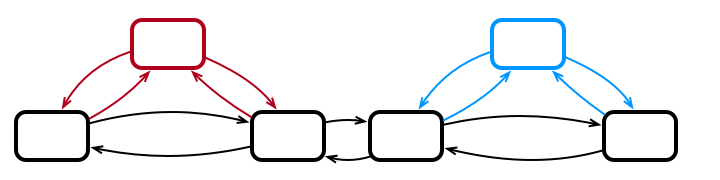Red Acquires Lock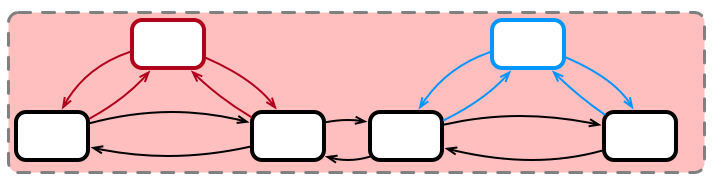Red Inserts Element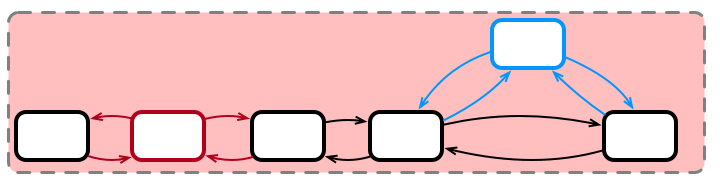Red Releases LockBlue Acquires Lock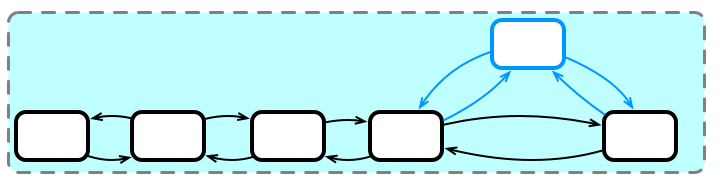Blue Inserts Element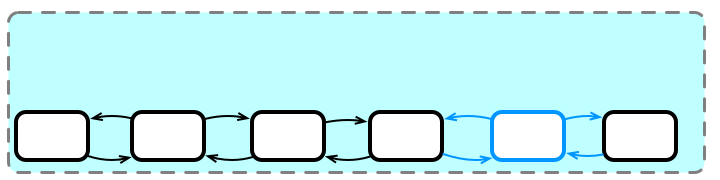Blue Releases Lock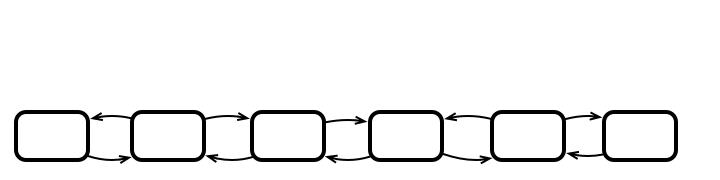…but…

…Could we Have Done Better?How?

What Should we Lock?

Not the whole list!

Idea: Locking Individual NodesWhich nodes need to be locked?

Locking Nodes in Code

class Node {
private Lock lock;
public Node next;
public Node prev;
public int value;

public void lock() { lock.lock(); }
public void unlock() { lock.unlock(); }
}

Insertion with Locked Nodes

public void insert (Node nd, value) {
Node cur = new Node(value);

nd.lock();
try {
Node next = nd.getNext();
if (next != null) next.lock();
nd.next = cur;
cur.prev = nd;
cur.next = next;
if (next != null) next.prev = cur;
} finally {
if (next != null) next.unlock();
nd.unlock();
}
}

Concurrent InsertionsAcquiring Locks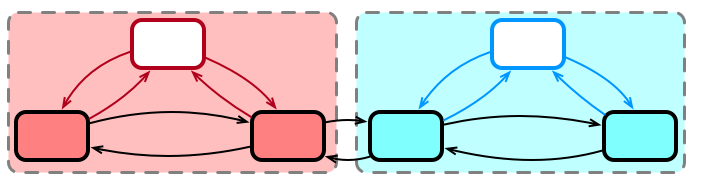Both Insert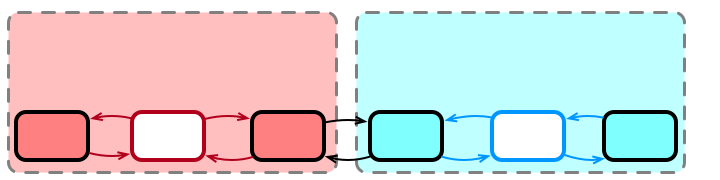Both ReleaseWhat Happens with Contention?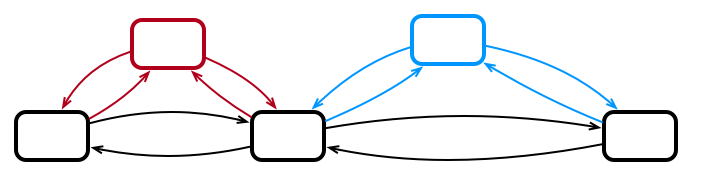Red Acquires Locks (Blue Waits)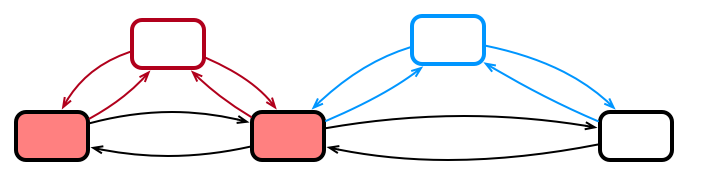Red Inserts & Releases Locks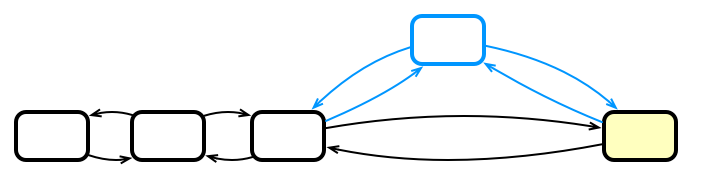Blue Finally Acquires Locks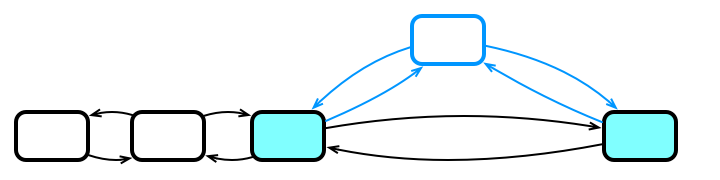Blue Inserts & Releases Locks